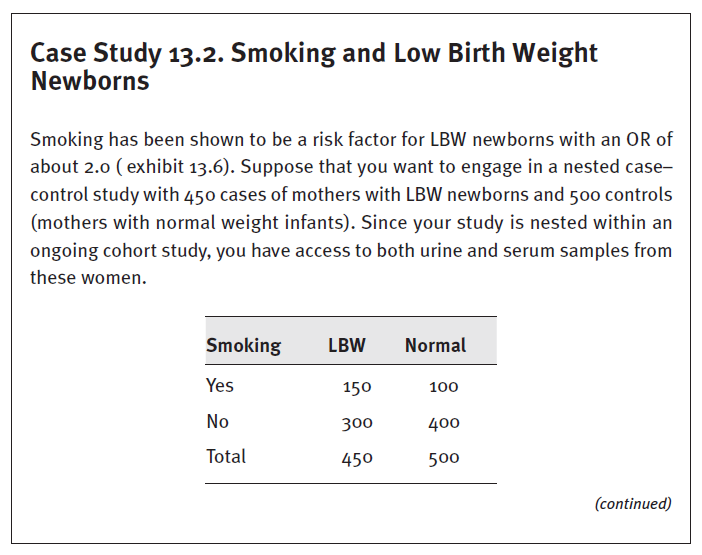Great Deal! Get Instant \$10 FREE in Account on First Order + 10% Cashback on Every Order Order Now

# Answer the following questions below and elaborate on your answer. 1. 1. Suppose that the true exposure status is 150 smokers among the cases and 100 smokers among the controls. What is the...1.
1.

Suppose that the true exposure status is 150 smokers among the cases and 100 smokers among the controls. What is the true crude OR?

2.
2.

What would be the observed OR using the serum thiocyanate measure for smoking status with a sensitivity of 86 percent and specificity of 79 percent?

3.
3.

So far we have been considering how the observed OR may be different from the true OR under conditions of an imperfect test to measure exposure. How might we do the opposite—adjust the observed OR to the true OR, taking into consideration the characteristics of the test of exposure?

Answered 1 days After Apr 28, 2023

## Solution

Ayan answered on Apr 30 2023
WRITTEN ASSIGNMENT        4
WRITTEN ASSIGNMENT
Question 1    3
Question 2    3
Question 3    4
References    5
Question 1
In the first question, we are asked to determine the genuine crude odds ratio (OR) based on the proportion of cases and controls that are smokers. An indicator of the relationship between exposure and disease outcome is the OR. The chances of exposure among cases in comparison to the odds of exposure among controls are disclosed (Olafimihan, Ariba & Malomo, 2022). We must first determine the probabilities of exposure in each group in order to compute the OR. In this instance, we are informed that 100 smokers make up the controls and 150 smokers make up the cases. Given that there are 350 instances overall, and that smoking is the exposure of interest, the probabilities of exposure among cases would be 150/350, which is equal to 0.43. Similarly, given there are 650 controls in total, the probabilities of exposure among controls would be 100/650, which may be expressed as 0.15. The real crude OR is 2.87 when we divide 0.43 by 0.15. This indicates that patients are 2.87 times more likely than controls to have...
SOLUTION.PDF